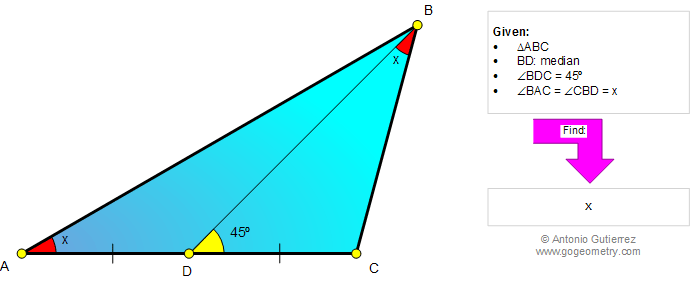###### Online Geometry Problem 986: Triangle, Median, Midpoint, Equal Angles, 45 Degrees. Level: High School, College, Mathematics Education

< PREVIOUS PROBLEM  |  NEXT PROBLEM >

 The figure below shows a triangle ABC with the median BD such that angles A and DBC are equal. If angle BDC is 45 degrees, find the measure of angle A. Try to use elementary geometry (Euclid's Elements.)Home | Search | Geometry | Problems | All Problems | Open Problems | Visual Index | 10 Problems | 981-990 | Triangles | Median | Midpoint | Congruence | 45 degrees | Email | Solution / comment | By Antonio Gutierrez